A polyhedron is a polyisohedron if its faces can be grouped into sets that are equivalent.

### Definitions:

Polyface:
A set of faces (aka polyside).
Polyisohedron:
A polyhedron where every polyface is equal and has the same relationship to every other polyface. The polyfaces are all disjoint.
Order of a polyisohedron:
The number of faces in a polyface. (Thus an isohedron is a polyisohedron of order 1.)

As an example, consider a Johnson solid with an entertaining name, the gyrobifastigium. It has 4 equilateral triangle faces and 4 square faces. You can pair up each triangle with a square so you have 4 polyfaces. Here’s one way to arrange them into congruent polyfaces, creating an order 2 polyisohedron with 4 polyfaces: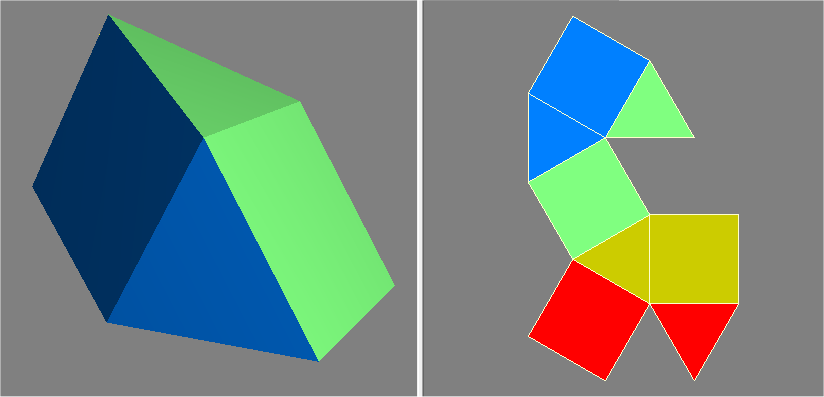Here's a second way to create congruent polyfaces: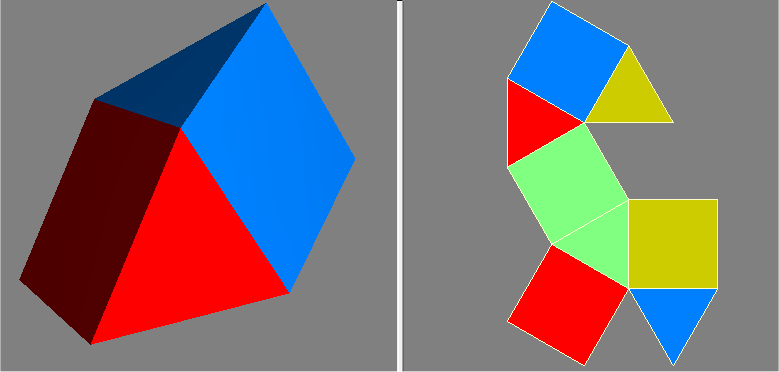With this next way of pairing up the triangles and squares, the 4 polysides aren’t congruent, hence it isn't polyisohedral: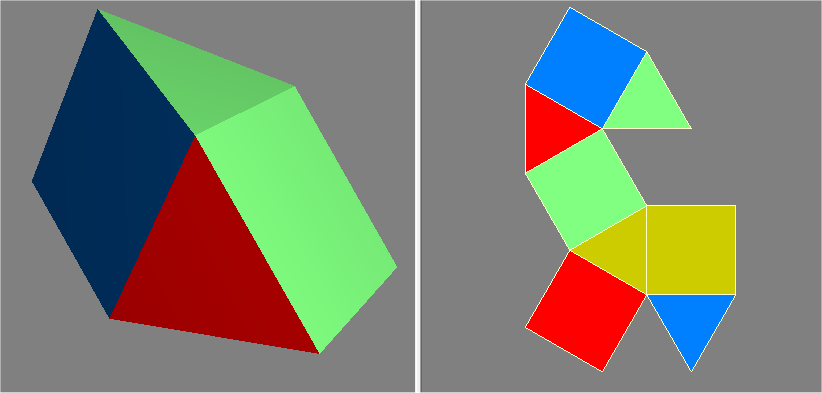### Lat-long polyisohedron

A lat-long polyisohedron uses a generalization of latitude and longitude on a sphere. To make one, start with a series of connected line segments. Create multiple equally spaced copies around the axis that connects the start and end point of the segments. The following example shows an order 4 lat-long polyishedron with 5 polyfaces.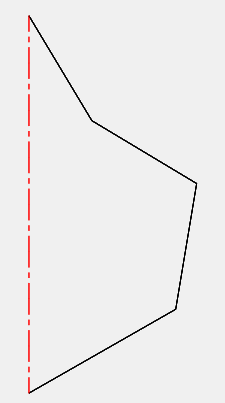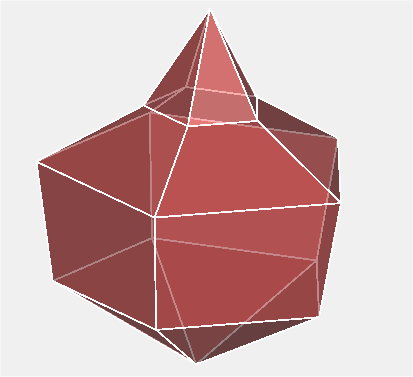If there are m line segments and n copies, the result will be an order m polyisohedron with n polysides. Each polyside will consist of two isosceles triangles and m-2 trapezoids.

The lat-long polyisohedron construction demonstrates that polyisohedra of any order 2 and greater exists with any number of sides 3 and greater. In contrast, order 1 polyisohedra, the isohedra, can't have an odd number of sides. They can have any number of even sides 4 and greater.

### Other examples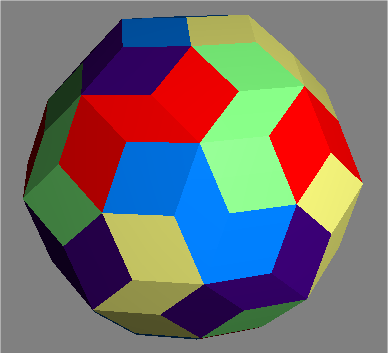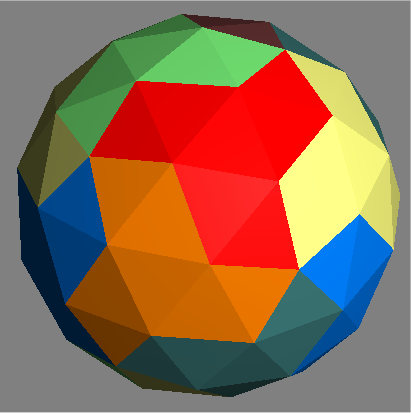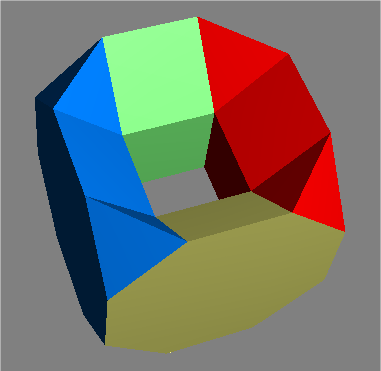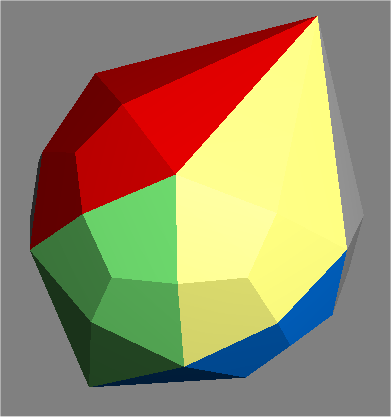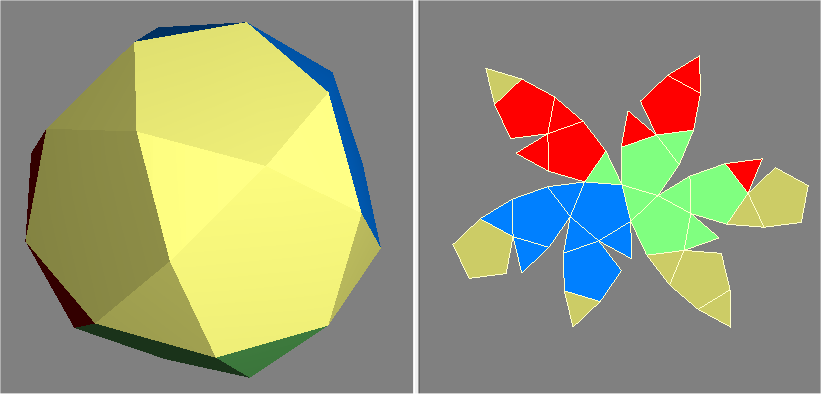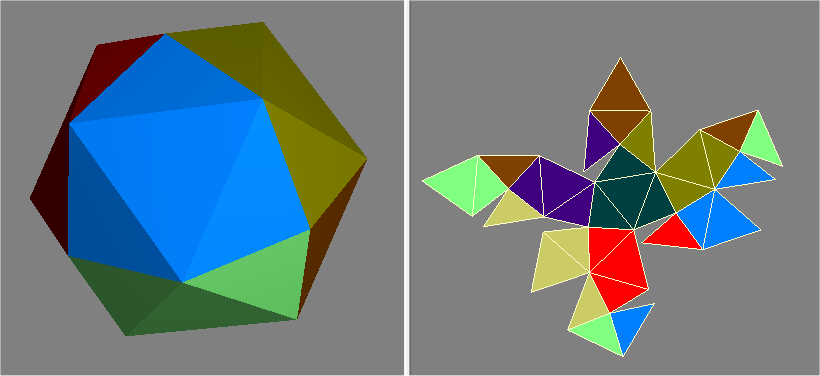Fair dice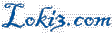© Scott Sherman 2010-2012 send comments to comments at this domain